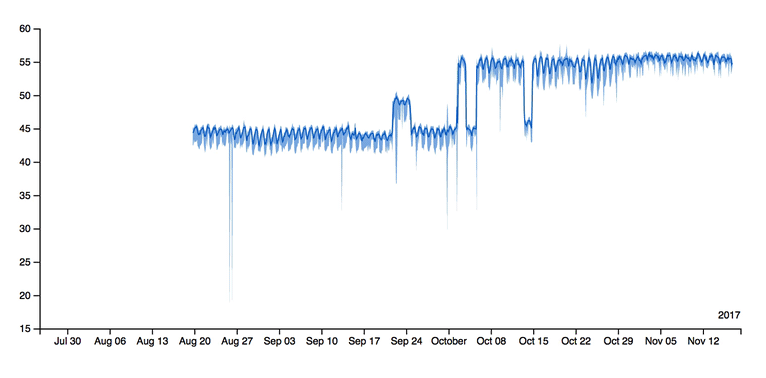BTrDB Explained

The Berkeley Tree DataBase (BTrDB) is pronounced "Better DB".

# BTrDB Explained

A next-gen timeseries database for high-precision, high-sample-rate telemetry.

Problem: Existing timeseries databases are poorly equipped for a new generation of ultra-fast sensor telemetry. Specifically, millions of high-precision power meters are to be deployed throughout the power grid to help analyze and prevent blackouts. Thus, new software must be built to facilitate the storage and analysis of its data.

Baseline: We need 1.4M inserts/s and 5x that in reads if we are to support 1000 micro-synchrophasors per server node. No timeseries database can do this.

## Summary

Goals: Develop a multi-resolution storage and query engine for many 100+ Hz streams at nanosecond precision—and operate at the full line rate of underlying network or storage infrastructure for affordable cluster sizes (less than six).

Developed at Berkeley, BTrDB offers new ways to support the aforementioned high throughput demands and allows efficient querying over large ranges.

Measured on a 4-node cluster (large EC2 nodes):

• 53 million inserted values per second
• 119 million queried values per second

Fast analysis

In under 200ms, it can query a year of data at nanosecond-precision (2.1 trillion points) at any desired window—returning statistical summary points at any desired resolution (containing a min/max/mean per point).High compression

Data is compressed by 2.93x—a significant improvement for high-precision nanosecond streams. To achieve this, a modified version of run-length encoding was created to encode the jitter of delta values rather than the delta values themselves. Incidentally, this outperforms the popular audio codec FLAC which was the original inspiration for this technique.

Efficient Versioning

Data is version-annotated to allow queries of data as it existed at a certain time. This allows reproducible query results that might otherwise change due to newer realtime data coming in. Structural sharing of data between versions is done to make this process as efficient as possible.

## The Tree Structure

BTrDB stores its data in a time-partitioned tree.

All nodes represent a given time slot. A node can describe all points within its time slot at a resolution corresponding to its depth in the tree.

The root node covers ~146 years. With a branching factor of 64, bottom nodes at ten levels down cover 4ns each.

levelnode width
12^62 ns (~146 years)
22^56 ns (~2.28 years)
32^50 ns (~13.03 days)
42^44 ns (~4.88 hours)
52^38 ns (~4.58 min)
62^32 ns (~4.29 s)
72^26 ns (~67.11 ms)
82^20 ns (~1.05 ms)
92^14 ns (~16.38 µs)
102^8 ns (256 ns)
112^2 ns (4 ns)

A node starts as a vector node, storing raw points in a vector of size 1024. This is considered a leaf node, since it does not point to any child nodes.

``````┌─────────────────────────────────────────────────────────────────┐
│                                                                 │
│                           VECTOR NODE                           │
│                     (holds 1024 raw points)                     │
│                                                                 │
├─────────────────────────────────────────────────────────────────┤
│ . . . . . . . . . . . . . . . . . . . . . . . . . . . . . . . . │ <- raw points
└─────────────────────────────────────────────────────────────────┘``````

Once this vector is full and more points need to be inserted into its time slot, the node is converted to a core node by time-partitioning itself into 64 "statistical" points.

``````┌─────────────────────────────────────────────────────────────────┐
│                                                                 │
│                            CORE NODE                            │
│                   (holds 64 statistical points)                 │
│                                                                 │
├─────────────────────────────────────────────────────────────────┤
│ ○ ○ ○ ○ ○ ○ ○ ○ ○ ○ ○ ○ ○ ○ ○ ○ ○ ○ ○ ○ ○ ○ ○ ○ ○ ○ ○ ○ ○ ○ ○ ○ │ <- stat points
└─┼─┼─┼─┼─┼─┼─┼─┼─┼─┼─┼─┼─┼─┼─┼─┼─┼─┼─┼─┼─┼─┼─┼─┼─┼─┼─┼─┼─┼─┼─┼─┼─┘
▼ ▼ ▼ ▼ ▼ ▼ ▼ ▼ ▼ ▼ ▼ ▼ ▼ ▼ ▼ ▼ ▼ ▼ ▼ ▼ ▼ ▼ ▼ ▼ ▼ ▼ ▼ ▼ ▼ ▼ ▼ ▼  <- child node pointers``````

A statistical point represents a 1/64 slice of its parent's time slot. It holds the min/max/mean/count of all points inside its time slot, and points to a new node holding extra details. When a vector node is first converted to a core node, the raw points are pushed into new vector nodes pointed to by the new statistical points.

levelnode widthstat point widthtotal nodestotal stat points
12^62 ns (~146 years)2^56 ns (~2.28 years)2^0 nodes2^6 points
22^56 ns (~2.28 years)2^50 ns (~13.03 days)2^6 nodes2^12 points
32^50 ns (~13.03 days)2^44 ns (~4.88 hours)2^12 nodes2^18 points
42^44 ns (~4.88 hours)2^38 ns (~4.58 min)2^18 nodes2^24 points
52^38 ns (~4.58 min)2^32 ns (~4.29 s)2^24 nodes2^30 points
62^32 ns (~4.29 s)2^26 ns (~67.11 ms)2^30 nodes2^36 points
72^26 ns (~67.11 ms)2^20 ns (~1.05 ms)2^36 nodes2^42 points
82^20 ns (~1.05 ms)2^14 ns (~16.38 µs)2^42 nodes2^48 points
92^14 ns (~16.38 µs)2^8 ns (256 ns)2^48 nodes2^54 points
102^8 ns (256 ns)2^2 ns (4 ns)2^54 nodes2^60 points
112^2 ns (4 ns)2^60 nodes

The sampling rate of the data at different moments will determine how deep the tree will be during those slices of time. Regardless of the depth of the actual data, the time spent querying at some higher level (lower resolution) will remain fixed (quick) due to summaries provided by parent nodes.

...